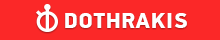GMAT Question of the Day - Daily to your Mailbox; hard ones only

 It is currently 26 Jun 2019, 03:22GMAT Club Daily Prep

Thank you for using the timer - this advanced tool can estimate your performance and suggest more practice questions. We have subscribed you to Daily Prep Questions via email.

Customized
for You

we will pick new questions that match your level based on your Timer History

Track

every week, we’ll send you an estimated GMAT score based on your performance

Practice
Pays

we will pick new questions that match your level based on your Timer History

Not interested in getting valuable practice questions and articles delivered to your email? No problem, unsubscribe here.If x, y, and z satisfy the inequalities shown, what is the least possi

Author Message
TAGS:

Hide Tags

Math ExpertV
Joined: 02 Sep 2009
Posts: 55802
If x, y, and z satisfy the inequalities shown, what is the least possi  [#permalink]

Show Tags00:00

Difficulty:45% (medium)

Question Stats:64% (01:42) correct36% (01:53) wrongbased on 167 sessions

HideShow timer Statistics6 ≤ |x| ≤ 8

1 ≤ |y| ≤2

3 ≤ |z| ≤ 4

If x, y, and z satisfy the inequalities shown, what is the least possible value of |x+y+z| ?

A. 0
B. 1
C. 2
D. 3
E. 4

_________________
CEOP
Joined: 18 Aug 2017
Posts: 3935
Location: India
Concentration: Sustainability, Marketing
GPA: 4
WE: Marketing (Energy and Utilities)
Re: If x, y, and z satisfy the inequalities shown, what is the least possi  [#permalink]

Show Tags

Bunuel wrote:
6 ≤ |x| ≤ 8

1 ≤ |y| ≤2

3 ≤ |z| ≤ 4

If x, y, and z satisfy the inequalities shown, what is the least possible value of |x+y+z| ?

A. 0
B. 1
C. 2
D. 3
E. 4

GMATinsight :

Sir how to solve this question ?

least values for x can be 6 , y=1 and z 3 ,
Is there a (-) sign missing from the given expressions?
_________________
If you liked my solution then please give Kudos. Kudos encourage active discussions.
InternB
Joined: 12 Mar 2018
Posts: 49
Location: Iran (Islamic Republic of)
GPA: 3.64
WE: Management Consulting (Energy and Utilities)
If x, y, and z satisfy the inequalities shown, what is the least possi  [#permalink]

Show Tags

2
1
Archit3110 wrote:
Bunuel wrote:
6 ≤ |x| ≤ 8

1 ≤ |y| ≤2

3 ≤ |z| ≤ 4

If x, y, and z satisfy the inequalities shown, what is the least possible value of |x+y+z| ?

A. 0
B. 1
C. 2
D. 3
E. 4

Sir how to solve this question ?

least values for x can be 6 , y=1 and z 3 ,
Is there a (-) sign missing from the given expressions?

Dear Archit3110
Notice that possible values of x lies in: -8≤x≤-6 & 6≤x≤8. So we can conclude that -2≤y≤-1 & 1≤y≤2 and, -4≤z≤-3 & 3≤z≤4.
So, least possible value for |x+y+z| might be calculated as x=-6, y=2 & z=4, which equals 0.
ManagerB
Joined: 29 May 2017
Posts: 128
Location: Pakistan
Concentration: Social Entrepreneurship, Sustainability
Re: If x, y, and z satisfy the inequalities shown, what is the least possi  [#permalink]

Show Tags

Bunuel

InternJoined: 15 Aug 2018
Posts: 2
Re: If x, y, and z satisfy the inequalities shown, what is the least possi  [#permalink]

Show Tags

Can someone provide the solution??
InternB
Joined: 12 Mar 2018
Posts: 49
Location: Iran (Islamic Republic of)
GPA: 3.64
WE: Management Consulting (Energy and Utilities)
If x, y, and z satisfy the inequalities shown, what is the least possi  [#permalink]

Show Tags

Jhim123 wrote:
Can someone provide the solution??

We are going to find such values for x, y & z that their absolute sum will be minimum. Since any absolute value is equal or greater than zero.
As available ranges we can consider x=-6, y=2 & z=4, so that sum will be zero (minimum value).If x, y, and z satisfy the inequalities shown, what is the least possi   [#permalink] 05 Dec 2018, 04:31
Display posts from previous: Sort by

If x, y, and z satisfy the inequalities shown, what is the least possi